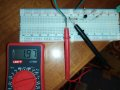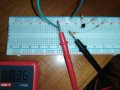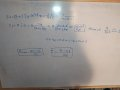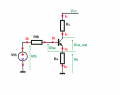# Constant current source does not work. What did I do wrong?

Thread Starter

#### babaliaris

Joined Nov 19, 2019
55I tried to create the circuit above but when I tested it, the current across the load was different for different loads. Is this expected? If it is, it seems weird because I thought the current is independent of the load since this is a constant current source.

VCC (Red Line on the breadboard) is 5VDC

Here I'm trying with load an 1kΩ resistor, the current is 1.97mA:As you see the transistor in the picture, the pins from left to right are controller, base, and emitter. R1 and R2 on the bias are 100Ω and Re is 1K.
The transistor has a b=420 (Model is 547C).

Then I changed the load from 1KΩ to 10ΚΩ and the current was 0.36mA:In the second picture, it seems like the load (10kΩ) goes into the base, but it is connected to the collector (First pin from the Left).

Did I do something wrong?

As always, thank you#### Jony130

Joined Feb 17, 2009
5,227
Without any load resistance at the collector, the collector current should be around Ic = (2.5V - 0.6V)/1k = 1.9mA.
The voltage at emitter is almost 2V, so to stay in the active region the load resistance cannot be larger than R = (5V - 2V)/1.9mA = 1.5kΩ

Therefore any load resistance large then 1.5kΩ will cause that the BJT will enter the saturation region. And in saturation, you no longer have a constant current source.

#### crutschow

Joined Mar 14, 2008
26,841

#### ronsimpson

Joined Oct 7, 2019
1,166
What Jony130 and Crutschow said.
In picture #2, if you move your black meter probe right to the other side of the 10k, the meter will read 1.9mA.
It will take 20V to get 2mA in a 10k resistor. You don't have 20V.

Thread Starter

#### babaliaris

Joined Nov 19, 2019
55
Oh, I understand now, thanks!!!

#### dl324

Joined Mar 30, 2015
12,688
Then I changed the load from 1KΩ to 10ΚΩ and the current was 0.36mA
I suggest that you not use your DVM to measure current. It can perturb the circuit and give you erroneous readings.

Instead, you can measure the voltage drop across a resistor and calculate current using Ohm's Law.

#### crutschow

Joined Mar 14, 2008
26,841
I suggest that you not use your DVM to measure current. It can perturb the circuit and give you erroneous readings.
If you put the meter in series with the load resistor, the only significant effect will be to slightly reduce the maximum load resistance that will stay in the constant-current region.

#### Audioguru again

Joined Oct 21, 2019
2,882
Why are your base bias resistors only 100 ohms? Then their current is 25mA! Since the transistor has such a high beta then 33k or 47k can be used for less power supply current wasted.

Thread Starter

#### babaliaris

Joined Nov 19, 2019
55
Why are your base bias resistors only 100 ohms? Then their current is 25mA! Since the transistor has such a high beta then 33k or 47k can be used for less power supply current wasted.
I'm new to transistors, I haven't completely understood everything.

Thread Starter

#### babaliaris

Joined Nov 19, 2019
55
Without any load resistance at the collector, the collector current should be around Ic = (2.5V - 0.6V)/1k = 1.9mA.
The voltage at emitter is almost 2V, so to stay in the active region the load resistance cannot be larger than R = (5V - 2V)/1.9mA = 1.5kΩ

Therefore any load resistance large then 1.5kΩ will cause that the BJT will enter the saturation region. And in saturation, you no longer have a constant current source.
@Jony130 Hello! I'm trying to understand what you are saying, but I'm still not there yet. I don't know the formulas you are using but I tried to figure them out. By doing some math I concluded that:The problem is how do you know Vb and Vc? In order to calculate them, you need to know Ic, Ib, but you calculate Ic by knowing Vb??? How do you do that? No matter what I do, if I try to calculate Vb or Vc I have Ib and b or (b+1) in my way...

#### Jony130

Joined Feb 17, 2009
5,227
The problem is how do you know Vb and Vc? In order to calculate them, you need to know Ic, Ib, but you calculate Ic by knowing Vb??? How do you do that? No matter what I do, if I try to calculate Vb or Vc I have Ib and b or (b+1) in my way...
We can solve for Vb and Vc.
The simplest way is to use a Thevenin's theorem and solve fo Ie or Ib.

Here you have an example:
https://forum.allaboutcircuits.com/threads/transistor-base.81886/#post-585781

Vth = Vcc * R2/(R1 + R2) = 2.5V and Rth = R1||R2 = 50Ω

And now we can write:

Vth - Ib*Rth - Vbe - Ie*Re = 0

Additional we know that

Ie = (β+1)*Ib or Ib = Ie/(β+1)

Ic = β*Ib

So we can solve for Ie directly:

Vth - Ie/(β+1)*Rth - Vbe - Ie*Re = 0

Ie = (Vth - Vbe)/( Rth/(β+1) + Re) = (2.5V - 0.6V)/(50Ω/421 + 1kΩ) = 1.9mA

Therefore:

Vb = Ie*Re + Vbe = 1.9V + 0.6V = 2.5V
Ve = Ie*Re = 1.9mA*1kΩ = 1.9V

This calculation assumes that the BJT is working in an active region. And in this circuit, the BJT will stay in the active region until Vc > Ve.
In your circuit, we have Ve ≈ 2V So, to remain in the active region the collector resistance cannot be large then this:

Rc_max = (Vcc - Ve)/Ie = (5V - 1.9V)/1.9mA = 1.6kΩ

Why? Because in the active region BJT works as a current source.
https://forum.allaboutcircuits.com/...r-amp-doubts-and-questions.91084/#post-663195

Hence the Vc voltage can be found using KVL:

Vc = Vcc - Ic*Rc

For example, if Rc = 1kΩ we would have Vc = 5V - 1.9mA*1kΩ = 3.1V.

But for Rc = 10kΩ we got a strange result Vc = 5V - 1.9mA*1kΩ = -14V

This means that our assumption that the BJT is still in the active region is no longer true. This should not be a surprise because for Rc =10kΩ and Re = 1kΩ the maximum current that can flow will be equal Ic_max ≈ Vcc/(Rc + Re) = 5V/11kΩ ≈ 454μA
And because Ic > Ic_max the BJT will be in the saturation region. And in this mode of operation Ic = β*Ib don't hold anymore.

But the basics circuit laws are still holding true. For BJT we have Ie = Ib + Ic and Vc = Ve+Vce(sat) and Vce(sat) < 0.2V for small-signal BJT's.

Therefore to find a numerical solution we again use Thevenin's theorem and replace R1 and R2 with a single Rth resistor plus Vth voltage source.

Vth = Vcc * R2/(R1 + R2) = 2.5V and Rth = R1||R2 = 50Ω

And the situation will look like this:From KCL we know that:

Ie = Ib + Ic

and

Ib = (Vth - Vbe - Ve)/Rb

Ic = (Vcc - Vce(sat) - Ve)/Rc

Ie = Ve/Re

And solving this for Ve voltage we got:

$V_E = \left( \frac{V_{TH} - V_{BE} }{R_{TH}} + \frac{V_{CC} - V_{ce(sat)}}{R_C}\right)*R_{TH}||R_C||R_E = \left( \frac{2.5V - 0.7V }{50\Omega} + \frac{5V - 0.1V}{10k\Omega}\right)*50\Omega||10k\Omega||1k\Omega \approx 1.7V$

And the collector current is Ic_sat = (5V - 1.7V)/10kΩ = 0.33mA; Ie = 1.7V/1kΩ = 1.7mA; Ib = Ie - Ic = 1.4mA. and Vc ≈ Ve = 1.7V

Analyze this example very carefully, if you have any doubts just ask.

Last edited:
Thread Starter

#### babaliaris

Joined Nov 19, 2019
55
We can solve for Vb and Vc.
The simplest way is to use a Thevenin's theorem and solve fo Ie or Ib.

Here you have an example:
https://forum.allaboutcircuits.com/threads/transistor-base.81886/#post-585781

Vth = Vcc * R2/(R1 + R2) = 2.5V and Rth = R1||R2 = 50Ω

And now we can write:

Vth - Ib*Rth - Vbe - Ie*Re = 0

Additional we know that

Ie = (β+1)*Ib or Ib = Ie/(β+1)

Ic = β*Ib

So we can solve for Ie directly:

Vth - Ie/(β+1)*Rth - Vbe - Ie*Re = 0

Ie = (Vth - Vbe)/( Rth/(β+1) + Re) = (2.5V - 0.6V)/(50Ω/421 + 1kΩ) = 1.9mA

Therefore:

Vb = Ie*Re + Vbe = 1.9V + 0.6V = 2.5V
Ve = Ie*Re = 1.9mA*1kΩ = 1.9V

This calculation assumes that the BJT is working in an active region. And in this circuit, the BJT will stay in the active region until Vc > Ve.
In your circuit, we have Ve ≈ 2V So, to remain in the active region the collector resistance cannot be large then this:

Rc_max = (Vcc - Ve)/Ie = (5V - 1.9V)/1.9mA = 1.6kΩ

Why? Because in the active region BJT works as a current source.
https://forum.allaboutcircuits.com/...r-amp-doubts-and-questions.91084/#post-663195

Hence the Vc voltage can be found using KVL:

Vc = Vcc - Ic*Rc

For example, if Rc = 1kΩ we would have Vc = 5V - 1.9mA*1kΩ = 3.1V.

But for Rc = 10kΩ we got a strange result Vc = 5V - 1.9mA*1kΩ = -14V

This means that our assumption that the BJT is still in the active region is no longer true. This should not be a surprise because for Rc =10kΩ and Re = 1kΩ the maximum current that can flow will be equal Ic_max ≈ Vcc/(Rc + Re) = 5V/11kΩ ≈ 454μA
And because Ic > Ic_max the BJT will be in the saturation region. And in this mode of operation Ic = β*Ib don't hold anymore.

But the basics circuit laws are still holding true. For BJT we have Ie = Ib + Ic and Vc = Ve+Vce(sat) and Vce(sat) < 0.2V for small-signal BJT's.

Therefore to find a numerical solution we again use Thevenin's theorem and replace R1 and R2 with a single Rth resistor plus Vth voltage source.

Vth = Vcc * R2/(R1 + R2) = 2.5V and Rth = R1||R2 = 50Ω

And the situation will look like this:

View attachment 193712
From KCL we know that:

Ie = Ib + Ic

and

Ib = (Vth - Vbe - Ve)/Rb

Ic = (Vcc - Vce(sat) - Ve)/Rc

Ie = Ve/Re

And solving this for Ve voltage we got:

$V_E = \left( \frac{V_{TH} - V_{BE} }{R_{TH}} + \frac{V_{CC} - V_{ce(sat)}}{R_C}\right)*R_{TH}||R_C||R_E = \left( \frac{2.5V - 0.7V }{50\Omega} + \frac{5V - 0.1V}{10k\Omega}\right)*50\Omega||10k\Omega||1k\Omega \approx 1.7V$

And the collector current is Ic_sat = (5V - 1.7V)/10kΩ = 0.33mA; Ie = 1.7V/1kΩ = 1.7mA; Ib = Ie - Ic = 1.4mA. and Vc ≈ Ve = 1.7V

Analyze this example very carefully, if you have any doubts just ask.
Ok, it seems that I'm behind in theory so it makes sense. I just entered the chapter about Thevenin.
But I will consider this when I reach a higher knowledge because it definitely helped me a lot!
It actually made me figure out how to choose the resistors in such a way, so I will always be in active mode.

Similar threads